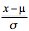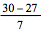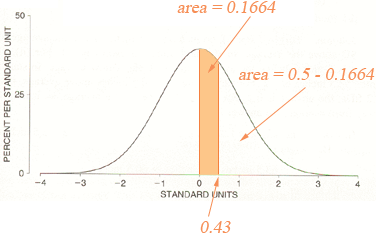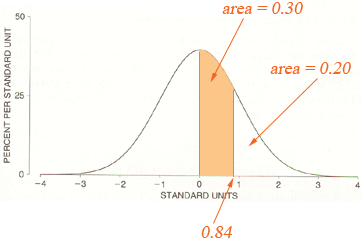SEARCH HOMEMath Central Quandaries & QueriesBecause of the relativity high interest rates, most consumers attempt to pay off their credits card bills promptly. However, this is not always possible. An analysis of the amount of interest paid monthly by a bank’s Visa cardholders reveals that the amount is normally distributed with a mean of $27 and a standard deviation of$7. What proportion of the bank’s Visa cardholder pay more than $30 in interest? What proportion of the bank’s Visa cardholder pay more than$40 in interest? What proportion of the bank’s Visa cardholder pay more than $15 in interest? What interest payment is exceeded by only 20% of the bank’s visa cardholder?Hi Jenny, The answer you sent for the first question was (a) P (Pay more than$30)                  = P (x > 30)
= P (>)
= P (Z > 0.43)
= 0.1664

I agree with everything except the last step. From the standard normal table P(0 < Z < 0.43) = 0.1664 and you want P(Z > 0.43) = P(0 < Z) - P(0 < Z < 0.43) = 0.5 - 0.1664 = 0.3336.You have a similar error in the second two parts. For the last question you want to find k so that P(X > k) = 0.20 which converts to P( (X - μ)/σ > (k - 27)/7) = P(Z > (k - 27)/7) = 0.20. Hence you want to find k so that P(0 < Z < (k - 27)/7) = 0.5 - 0.20 = 0.30.In my normal table the closest I can find to 0.30 is P(0 < Z < 0.84) = 0.2995 hence (k - 27)/7 = 0.84. Solve for k.

I hope this helps,
HarleyMath Central is supported by the University of Regina and The Pacific Institute for the Mathematical Sciences.